### Home > AC > Chapter 12 > Lesson 12.1.1 > Problem12-10

12-10.
1. Describe how you add and subtract fractions that have a common denominator. Homework Help ✎

1. Add or subtract the fractions below. Draw a diagram to show that your answer is correct.

1.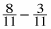2.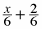2. What if you are given two fractions to add or subtract and the denominators are not the same? Add the fractions below and check your result on your calculator.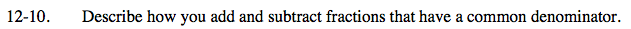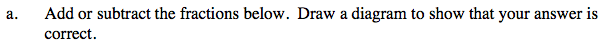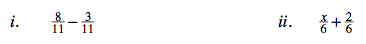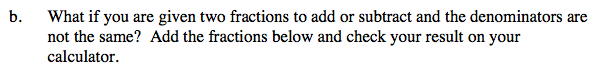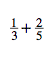To add or subtract fractions with a common denominator, add or subtract the numerators.

For part (b), you must first find a common denominator.